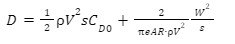# Calculating Drag

Hello,
I would like to ask if the Drag i get via the incompressive flow analysis, is about the total Drag of my body.
This is because i simulating a Wing, but the results i get in theory (calculating the parasitic Drag+ the induced Drag) are differnet fron that on the software …So, can you tell me please how do you calculate the total Drag, to compare it with my theory?

Hello kkatsaflioglou,

thanks for reaching out to us.

i think there is a misunderstanding about how the drag in the CFD solution is calculated.
The difference between the CFD solution and the theoretical value is that in the CFD we solve the complete flow field around the wing, whilst in the theory, we have to take each component into account.(e.g. pressure-induced drag, shear force, etc.)
Since the hole flow field is calculated all of these can be taken into account. Therfore we can calculate the drag force based on the pressure and WSS) Thus the force you get from the CFD solution will be the total drag.
Can you please share your calculation and the cfd case with us so that we can compare these two.

Best regards
Sebastian

This is my Wing Project with the follow specs

• Wing Area: 1,182m^2

• Wing span: 3,430 m

• Aspect Ratio: 10

*Oswald factor e: 0,76

• Weight (Lift expectet— L=W): 323.73 N

• Speed : starting point is 25m/s…but the wing has leading edge Sweep angle Λ=4,5deg. (V=V0cosΛ=24,92m/s)

• at ρ=1,225kg/m^2

• NACA 4412 (zero Lift cd=0,01638 from airfoiltools.com at Re: 200.000)

• AoA=4

If i use the Total Drag equation :The Parasitic Drag = 10,60 N and the induced Drag=9,83 N, so the total Drag is: 20,23N
But via the simulation bellow, i get Drag = 11,15N

https://www.simscale.com/workbench/?pid=812182538935247774&mi=spec%3A8189a511-d4d4-46ae-b817-f2d0091b044c%2Cservice%3ASIMULATION%2Cstrategy%3A3&ps=analysis%2FboundaryConditions%2F0

Hello @kkatsaflioglou , thanks for sharing these information. Sebastian already made some great points. I’ll also have a few comments according to latest info you just shared.

-First of all, weight and inertia are never included in static CFD simulations such as this one. Weight can only be used to predict lift coefficient in early design stages.

-The website (airfoiltools) that you’re referring is using a panel code called XFoil to obtain aerodynamic coefficients. Panel codes are not the most accurate tools when you have seperation zones, chaotic flows comparing with CFD and experimental methodologies. Viscous effects can not be properly included in the computation, consequently they usually give relatively accurate results in lift coefficients but not in drag coefficients.

-With a rough calculation, Reynold’s number around your wing is about 800,000 but you’re referring to 200,000. Choosing a higher Reynolds number from the graphs in website will give you smaller drag coefficients.

-Angle of attack is not 4 in your CFD simulation but 0. You can set an angle of attack by using flow directions in your velocity inlet boundary condition.

After tuning these settings, you should obtain closer values to your reference. However, please be aware that the methodologies you present here are mostly empirical formulations so, you should expect slightly different results while comparing with high-fidelity CFD results.

Kaan.

Thank you for the support

-i only get the zero-lift cd from airfoiltools. com…in case that the true zero-lift drag is different…that does not provide such a difference in the total Drag.
-I set the AoA in the CAD mode…setting the rotation in z-axis

Zero-lift Cd (Cd_0) only assumes an infinite wing meaning that effects of vortices near the wing tip will not be counted due to lift or pressure difference between both sides. Cd_0 still consists of drag due to pressure and drag due to shear, and these values may not be very accurate while using panel codes as I mentioned earlier. Correcting the Reynold’s number should still give you better/closer results though.

Also thanks for the headsup regarding the angle of attack, then angle of attack shouldn’t be a problem.

I set a new (smaller) zero-lift cd in the parasatic drag (1/2 ρ V^2 Cd_0 s) but aggain the results are much bigger…what im doing wrong? i know that the equation i used is not supper accurate but aggain it is a lot of difference between my calclulation and the CFD results.

What is the resultant total drag you get right now?

I also realized that you don’t include the viscous force in x direction to your total drag sum which is around 2.91 N.

oh, i forget it…my theoretical resaults are 15,15N…now the resaults from simulation are 11,15+2,91=14,06N.
I think now we are pretty close

Great! Rest of the difference may be due to several factors really such as: the fidelity of the tools, oswald efficiency, etc.

But in general I would say that it’s a pretty successful comparison with theoretical values.
Best,
Kaan.

2 Likes

Thank you a LOT…you are great!!!

2 Likes